BCA 2nd sem Data Structure using C paper 2017

UniversityGGSIPU, New Delhi
CourseBCA
Semester2
SubjectData Structure using C
Year2017
Uploaded By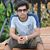Question Marks 3 requests (a) Add and subtract the following two sparse matrices.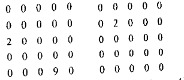This question has 0 answers so far. 5 1 requests (b) Perform insertion sort on the following values.6, 55, 11, 10, 18  This question has 0 answers so far. 5 0 requests (c) Convert the following infix expression into postfix expression.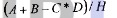This question has 0 answers so far. 5 0 requests (d) Write the preorder traversal of the following tree.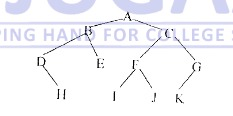This question has 0 answers so far. 5 0 requests (e) Write a Recursive function to count number of nodes in Tree. This question has 0 answers so far. 5 0 requests (a) Classify primitive and non-primitive data structures. Discuss the operations performed on data structures.  This question has 0 answers so far. 6 0 requests (b) Evaluate the following postfix expression using stacks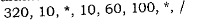This question has 0 answers so far. 6.5 0 requests (a) Explain why circular queue is better than linear queue?  This question has 0 answers so far. 6 0 requests (b) Discuss D-queues and priority queues. What are the applications stacks and queues?  This question has 0 answers so far. 6.5 0 requests (a) Write a function to insert a node at the end of single linked list. This question has 0 answers so far. 6 0 requests (b) Write a function to delete a node from beginning of double link list. This question has 0 answers so far. 6.5 0 requests (a) A binary tree T has 09 nodes. The inorder and preorder traversals of T yield the following sequences of nodes.  Inorder: D G B A H E I C F Preorder: A B D G C E H I F Draw the tree T  This question has 0 answers so far. 6 0 requests (b) Consider the following binary tree T with N-10 nodes. What is the inorder traversal of the tree?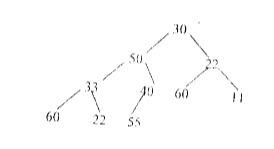This question has 0 answers so far. 6.5 0 requests (a) Construct B-tree of order 3 by inserting the following keys in the order shown.          18 19. 6, 10, 40  This question has 0 answers so far. 6.5 0 requests (b) Construct Binary Search Tree of the following keys in the order shown               1, 2, 3, 15, 8, 25, 7, 9, 10, 13  This question has 0 answers so far. 6 0 requests (a) Construct an AVL search tree of the following values                    11, 20, 23, 5,3  This question has 0 answers so far. 6 1 requests (b) Insert the following values in the order of their occurrence 30, 31 in the given B tree of order 5.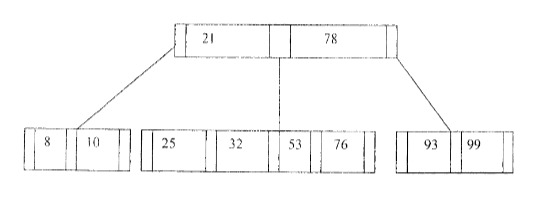This question has 0 answers so far. 6.5 0 requests (a) Define hashing. Why do we use hashing? Discuss any two hashing methods with example, This question has 0 answers so far. 6.5 0 requests (b) Which searching technique is best and under what conditions?Justify your answer with the help of an example. This question has 0 answers so far. 6 0 requests (a) Compare Selection sort and Merge sort. This question has 0 answers so far. 6 0 requests (b) Which sorting technique is better and why? Explain with an example. This question has 0 answers so far. 6.5